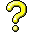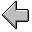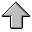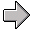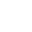Functional Programming5. Definition and Evaluation

## 5.1 Evaluation Strategies

An evaluation strategy is a set of rules for evaluating expressions. Evaluation strategies are important in expressions, for example (1-x)(1+x) when x is very close to 1 might be better evaluated as 1-2x-x*x. Where evaluation strategies are important, say in numerical computation, we need to know a lot about how the compiler works.

In a functional language, evaluation strategies focus on how the function applications are processed. An evaluation strategy asnwers the questions: What order are the arguments evaluated? When are they evaluated? What form does evaluation take?

Because there are no side-effects in a pure functional language, evaluation takes the form of rewriting expressions using the conversion rules to reduce the expression to be as "small" as possible.

Applicative order means to evaulate the arguments of a function application in left-to-right order, exactly as you would do if you were required to Curry (programming language) the function.

When we compute a function we usually assume that all arguments have a value before the function evaluation begins. Strict evaluation (or eager evaluation) means to evaluate all the arguments to a function before evaluating the function. Applicative order evaluation is strict. But not all strict evaluation need be in applicative order.

In non-strict evaluation arguments need not be evaluated until they are actually required. Lazy evaluation is a form of non-strict evaluation in which arguments are not evaluated until required.

A computation that does not terminate under strict evaluation, can terminate under lazy evaluation. For example, the function that produces an infinite list of integers that is then used in a function that only requires the first 3 elements of the list.

Normal order means to evaluate the arguments of a function only when needed. Normal order reduction always chooses to reduce first the leftmost expression. After one reduction step, it chooses to do the leftmost expression again. }

In the λ-calculus, all reduction orders that terminate give the same result. That is, the λ-calculus is confluent, or has the Church-Rosser property. What this means is that changing the evaluation strategy only changes whether the program terminates or not, not the final result of the computation. The invariance of the final result of a terminating computation clearly need not hold in the case when we have side-effects.

Note: Scheme is dynamically typed. It's data has types (like integer and string), it's just that the types of arguments to functions are not generally known at compile time, only at evaluation time.

## 5.2 Special Forms Are Lazy

Scheme uses applicative order eager evaluation. This means that, in general, the arguments of an application are evaluated first in left to right order, and then their values are passed as arguments to the function.

If all the arguments must be evaluated first, this can be problematic, for example when selecting the true or false case of an `if` operation. Consider the case where you need to guard against division by zero: ``` (if (not (= x 0)) (something (/ 1 x)) (error "bad x")) ``` This means that certain constructs in Scheme that look like normal function application are not. The main examples are `if, and, or, lambda`.
• `if` expressions are special because they only evaluate one of the then/else cases;
• `and` `or` are special because they use short-circuit evaluation stopping the moment the value of the expression is decided
• `lambda` is special because it not only builds code to be evaluated in the future, it also preserves the lexical context of all the free variables in the lambda expression.
Interestingly, only a few special forms are necessary, the rest can be implemented from them - in fact all one needs is `lambda`.

code/LambdaScheme/SpecialForms.rkt

 `    ``#lang racket` `    `` ` `    ``; when does a term get evaluated?` `    `` ` `    ``(define X 2)` `    ``(define Y 3)` `    `` ` `    ``; if the world was purely functional it wouldn't matter if both parts` `    ``; of an 'if' were evaluated beforehand.  Although it could be quite costly` `    ``; since you are doing unnecessary work that is just thrown away.` `    `` ` `    ``(if (< X Y)` `    ``    (+ X X)` `    ``    (* Y Y)` `    ``    )` `    `` ` `    ``; but if there are side effects it does matter` `    ``(list (< X Y)` `    ``    (print "true")` `    ``    (print "false")` `    ``    )` `    `` ` `    `` ` `    ``; so 'if' is clearly a different kind of operation than, say, 'list'` `    ``(if (< X Y)` `    ``    (print "true")` `    ``    (print "false")` `    ``    )` `    `` ` `    ``; note that the and and or operations are lazy, so an if ` `    ``;   (if condition consequent alternate) ` `    ``; can also be written as this:` `    ``;   (or (and condition consequent) alternate)` `    ``(or (and (< X Y)` `    ``         (+ X X)` `    ``    )` `    ``    (* Y Y)` `    ``    )` `    `` ` `    ``(or (and (< X Y)` `    ``         (print "true")` `    ``    )` `    ``    (print "false")` `    ``    )` `    `` ` `    ``; if we have and/or we could define our own if functon using` `    ``; the above relationship.  ` `    `` ` `    ``; But the arguments to our new function get evaluated before the call` `    ``(define my-if` `    ``  (lambda (condition consequent alternate)` `    ``    (or (and condition consequent ) alternate )` `    ``    )` `    ``  )` `    `` ` `    ``; it doesn't matter here` `    ``(my-if (< X Y)` `    ``    (+ X X)` `    ``    (* Y Y)` `    ``    )` `    `` ` `    ``; but it does if there are side effects in an argument!` `    ``(my-if (< X Y)` `    ``    (print "true")` `    ``    (print "false")` `    ``    )` `    `` ` `    ``; so we have to defer the evaluation of arguments in an ` `    ``; if by wrapping them in lambda, and then evaluating only` `    ``; the one selected by the condition.  Note the ( ) around` `    ``; the consequent and alternate closures to force evaluation` `    `` ` `    ``; Note that the operations or/and must also be special forms` `    ``; so somewhere there must be a built in conditional special` `    ``; form that delays evaluation of its arguments.` `    `` ` `    ``(define my-lazy-if` `    ``  (lambda (condition consequent-closure alternate-closure)` `    ``    (or (and condition (consequent-closure) ) (alternate-closure) )` `    ``    )` `    ``  )` `    `` ` `    ``; it doesn't matter here` `    ``(my-lazy-if (< X Y)` `    ``    (lambda () (+ X X))` `    ``    (lambda () (* Y Y))` `    ``    )` `    `` ` `    ``; but it does if there are side effects in an argument!` `    ``(my-lazy-if (< X Y)` `    ``    (lambda () (print "true"))` `    ``    (lambda () (print "false"))` `    ``    )` `    `` ` `    ``; lambda wrapping to create lazy evaluation is somewhat annoying.` `    ``(my-lazy-if` `    `` (< X Y)` `    `` (lambda ()` `    `` (my-lazy-if (> X Y) (lambda () "cc true") (lambda () "cc false")))` `    `` (lambda () "c false")` `    `` )` `    `` ` `    ``; that is why it is built in to the special forms like if, and, or` `    ``; using macros.` `    `` ` `    ``; we can also introduce special syntax that does the closure construction` `    ``; for us` `    ``(define-syntax-rule (macro-if condition consequent alternate)` `    ``  ((lambda (consequent-closure alternate-closure)` `    ``    (or (and condition (consequent-closure) ) (alternate-closure) )` `    ``    )` `    ``   (lambda () consequent)` `    ``   (lambda () alternate)` `    ``   )` `    ``  )` `    `` ` `    ``(macro-if #t (print 1) (print 0))` `    `` ` `    ``(define ifif (lambda (x)` `    ``   (macro-if (= x 0) (print "a") ` `    ``             (macro-if (= x 1) (print "b") (print "c")))))`

When code is wrapped inside a lambda expression it creates a lexical closure which contains not only the function but the referencing environment that provides references for all the free variables in the lambda expression at time of creation.

In the `if` examples above we use the closure of a parameterless function to delay the evaluation of code. Such a closure is called a thunk (functional programming). See http://docs.racket-lang.org/reference/procedures.html

## 5.3 Implementing Conditional Special Forms With Functional Truth

All of the above examples of lazy evaulation skirt around the issue of needing some feature in the language that is intrinsically lazy. We converted `if` into `and` and `or`, the latter of which are lazy. Thus we didn't avoid the issue of having a logical value control evaluation.

However, if you change the definition of true and false, then you can achieve lazy evaluation of conditionals by exploiting the behaviour of lambda expressions. More on racket macros can be found in http://docs.racket-lang.org/guide/pattern-macros.html.

code/LambdaScheme/lambda-logic.rkt

 `    ``#lang racket` `    ``(define TRUE (lambda (x y ) x))` `    ``(define FALSE (lambda (x y) y))` `    `` ` `    ``; with TRUE and FALSE defined this way, we actually can implement` `    ``; if without cheating.  Note how the logical value selects the ` `    ``; wrapping lambda to be evaluated, and then the evaluation occurs.` `    `` ` `    ``(define-syntax-rule (lif condition consequent alternate)` `    ``  ( (condition (lambda () consequent) (lambda () alternate)) ) )` `    `` ` `    ``; as well as the other logical operations.` `    ``(define-syntax-rule (land a b) (a b a))` `    `` ` `    ``(define-syntax-rule (lor a b) (a a b))` `    `` ` `    ``(define-syntax-rule (lnot a) (a FALSE TRUE))` `    `` ` `    ``(define-syntax-rule (lxor a b) (a (b FALSE TRUE) b))` `    `` ` `    ``(lxor TRUE TRUE)` `    ``(lxor TRUE TRUE)` `    ``(define a TRUE)` `    ``(define b FALSE)` `    ``(lif (land a b) (print "1") (print "0"))` `    `` ` `    `` ` `    ``; further exploting the macro capability we can introduce the ` `    ``; extended version of and and or that take 0 or more arguments and` `    ``; short-circuit evaluation` `    `` ` `    ``(define-syntax lland` `    ``  (syntax-rules ()` `    ``    [(lland) TRUE]  ; no arguments, TRUE is identity for and` `    ``    [(lland a)  a]` `    ``    [(lland a b ...) (a (lland b ...) FALSE) ]` `    ``    ))` `    `` ` `    ``(define-syntax llor` `    ``  (syntax-rules ()` `    ``    [(llor) FALSE]` `    ``    [(llor a)  a]` `    ``    [(llor a b ...) (a TRUE (llor b ...)) ]` `    ``    ))` `    `` ` `    ``(lland TRUE FALSE TRUE)`

## 5.4 Implementing Lazy Evaluation

The techniques above can be used to implement lazy evaluation. When lazy evaluation is explicit, the programmer has control over when an expression is to be evaluated. They can delay the evaluation of an expression, and then force it later when needed. Here is a simple implementation of weak delay and force using the wrapping techniques above.

code/LambdaScheme/w-delay-force-1.rkt

 `    ``#lang racket` `    `` ` `    ``; create some functions to track how often something` `    ``; is used.  It modifies a global state variable.` `    `` ` `    ``(define global-eval-count 0)` `    ``(define (reset-count) (set! global-eval-count 0))` `    ``(define (get-count) global-eval-count)` `    ``(define (inc-count)` `    ``  (set! global-eval-count (+ 1 global-eval-count))` `    ``  (displayln (format "eval count ~a" global-eval-count))` `    ``  )` `    `` ` `    ``; define syntax for weak delay of expr.  Returns a ` `    ``; closure that when evaluated evaluates expr in the ` `    ``; original context.` `    ``(define-syntax-rule (w-delay expr) ` `    ``  (lambda ()` `    ``    (inc-count)  ; for tracing purposes only` `    ``    expr` `    ``    ))` `    `` ` `    ``; define syntax for weak force of a delayed expr` `    ``; simply evaluates the lambda` `    ``(define-syntax-rule (w-force thunk)` `    ``  (thunk)` `    ``  )` `    `` ` `    ``(define (test-it)` `    ``  (reset-count)` `    ``  (define v1 (w-delay (+ 40 2)))` `    ``  (displayln (w-force v1))` `    ``  (displayln (w-force v1))` `    ``  ` `    ``  ` `    ``  ; f1 assumes that w-y is a delayed expression` `    ``  (define (f1 n w-y)` `    ``    (if (= n 0) 1 (+ (w-force w-y) 1)))` `    ``  ` `    ``  (define x 0)` `    ``  (display "on 0: ") (displayln (f1 0 (w-delay (/ 1 x))))` `    ``  ` `    ``  (display "on 1: ") (displayln (f1 1 (w-delay (/ 1 x))))` `    ``  )` `    ``  `
We say that `w-delay` and `w-force` are weak because they do not do anything fancy, like caching values after a force. This achieves call by name parameter passing.

code/LambdaScheme/w-delay-force-2.rkt

 `    ``#lang racket` `    ``; define syntax for weak delay of expr.  Returns a` `    ``; closure that when evaluated evaluates expr in the` `    ``; original context.` `    ``(define-syntax-rule (w-delay expr)` `    ``  (lambda () expr))` `    `` ` `    ``; define syntax for weak force of a delayed expr` `    ``; simply evaluates the lambda` `    ``(define-syntax-rule (w-force thunk)` `    ``  (thunk) )` `    `` ` `    ``; a double function that assumes its argument is w-delayed.` `    ``(define (double-w w-x)` `    ``  (+ (w-force w-x) (w-force w-x)))` `    `` ` `    ``; (w-double 42)` `    ``; (w-double (w-delay 42))` `    `` ` `    ``; a double function that w-delays its result` `    ``(define (w-double-w w-x)` `    ``  (w-delay (+ (w-force w-x) (w-force w-x)) ) )` `    `` ` `    ``; (define r (w-double-w (w-delay 42)))` `    ``; (w-force r)` `    `` ` `    `` ` `    ``; weak delay and force give you a kind of call by name behaviour,` `    ``; as if you substituted the parameter into the code, while avoiding` `    ``; any new lexical bindings.` `    `` ` `    ``; note how this breaks referential transparency ` `    `` ` `    ``; a global state changing operation` `    ``(define state 0)` `    ``(define (next-state)` `    ``  (set! state (+ 1 state))` `    ``  state)` `    `` ` `    ``(define v1 (w-delay (next-state)))` `    `` ` `    ``; every force gives a different value` `    ``; (w-force v1))` `    ``; (w-force v1))` `    `` ` `    ``; and so does double` `    ``; (double-w v1)` `    ``  ` `    ``; and things are really bad if you w-delay even further` `    ``(define v2 (w-double-w v1))` `    `` ` `    ``; state` `    ``; v2` `    ``; (w-force v2)` `    ``; (next-state)` `    ``; (w-force v2)` `    `` `

## 5.5 Diversion - Objects Are Just Closures

If we want to implement a caching type of lazy evaluation, then we need to preserve state information about the expression. The way to do this is to encapsulate the state information inside a closure, and the closure takes methods and performs operations. This is one way to implement object-based (no inheritance) encapsulation of state.

code/LambdaScheme/objects-1.rkt

 `    ``#lang racket` `    `` ` `    ``; the constructor for a counter object, returns a closure with` `    ``; the initial value of the counter set to init.` `    `` ` `    ``(define (create-counter init)` `    ``  (define counter init)` `    ``  ` `    ``  ; the lambda captures the local defintion of counter` `    ``  (lambda (op)` `    ``    (cond` `    ``      [ (eq? op 'get) counter ]` `    ``      [ (eq? op 'inc)` `    ``        (set! counter (+ 1 counter)) counter ]` `    ``      [ else (error (format "Invalid operation ~a" op) ) ]` `    ``      ))` `    ``  )` `    ``          ` `    ``; (define c (create-counter 43))` `    ``; (c 'get)` `    ``; (c 'inc)` `    ``; (c 'get)` `    ``; (c 'dec)`

Taking advantage of variable length argument lists, you can implement a full suite of methods.

code/LambdaScheme/objects-2.rkt

 `    ``#lang racket` `    `` ` `    ``; the constructor for a counter object, returns a closure with` `    ``; the initial value of the counter set to init.` `    `` ` `    ``(define (create-counter init)` `    ``  (define counter init)` `    ``  ` `    ``  ; the lambda captures the local defintion of counter` `    ``  (lambda args ` `    ``    ; if no param list, return the counter` `    ``    (if (null? args) counter` `    ``        (let ( [ op (first args) ] )` `    ``        (cond` `    ``          [ (eq? op 'get) counter ]` `    ``          [ (eq? op 'inc)` `    ``            (set! counter (+ 1 counter)) counter ]` `    ``          [ (eq? op 'inc-by)` `    ``            (set! counter (+ (second args) counter)) counter ]` `    ``          [ else (error (format "Invalid operation ~a" op ) ) ]` `    ``          ))` `    ``        )` `    ``  ))` `    `` ` `    ``; (define o1 (create-counter 0))` `    ``;` `    ``; (o1 'inc)` `    ``; (o1)` `    ``; (o1 'inc-by 2)` `    ``; (o1)`5. Definition and Evaluation Notes on Functional Programming / Version 2.10 2014-02-24